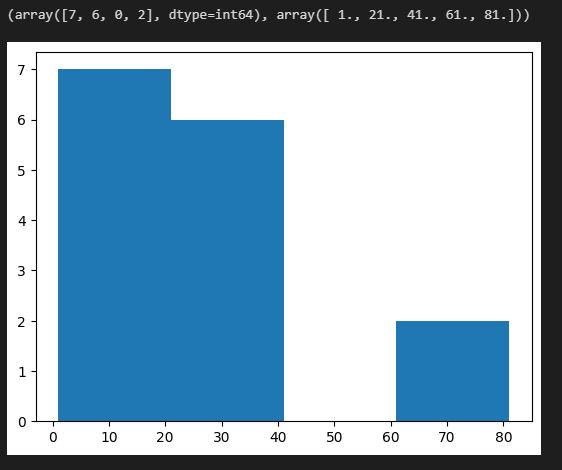# Grouped frequency distributions

Rating & reviews (0 reviews)
Study notes
Visual Studio - Jupyter notebook

Syntax:
numpy.histogram(a, bins=10, range=None, density=None, weights=None)

• numpy.histogram
• matplotlib.pyplot.hist
• matplotlib.pyplot.show()

The number of observations of each class interval of a variable. Class intervals are ordered groupings of a variable’s values.

# Grouped Frequency Distribution

import numpy as np
import matplotlib.pyplot as plt

x = [21, 2, 23, 4, 25, 6, 27, 81, 1, 32, 20, 4, 25, 62, 4]

# Group in 4 clases (range)
nb = 4

# compute
z= np.histogram(x,nb)

# show
plt.hist(x,nb)

print(z)
plt.show()

# Class (range) -> Nr of elementsin class
# 0 -> 2 1(21 not included) -> 7
# 21 - 41 (41 not included) -> 6
# 41 - 61 (61 not included) -> 0
# 61 - 81 (81 included) -> 2

Result:Main source:
numpy.histogram — NumPy v1.24 Manual